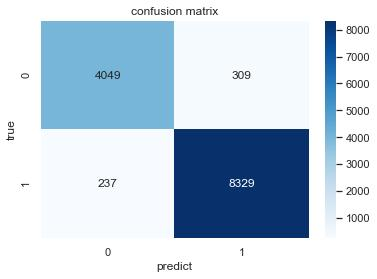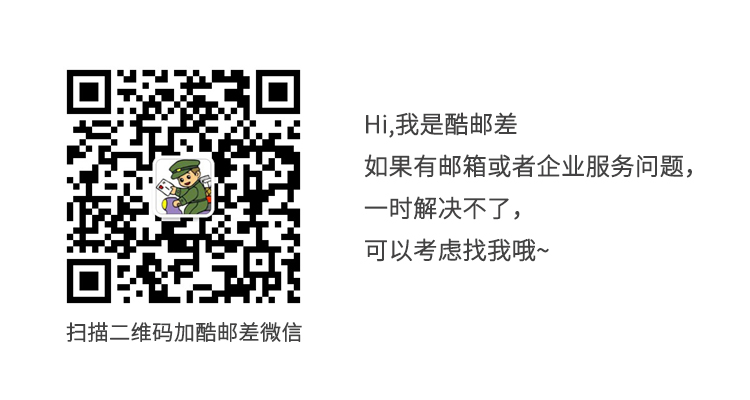### 朴素贝叶斯算法进行垃圾邮件识别

邮箱网  0条评论  1689次浏览  2022年03月10日 星期四 20:58

1. 提取正文部分的文本特征；

2. 划分训练集和测试集（可以借助工具包。一般笔记本就足够运行所有数据，认为实现困难或算力不够的同学可以采样一部分数据进行实验。）；

3. 使用朴素贝叶斯算法完成垃圾邮件的分类与预测，要求测试集准确率Accuracy、精准率Precision、召回率Recall均高于0.9（本次实验可以使用已有的一些工具包完成如sklearn）；

4. 对比特征数目（词表大小）对模型效果的影响；

5. 提交代码和实验报告。

1. 邮件头信息有时也可以协助判断垃圾邮件，欢迎学有余力的同学们尝试；

2. 尝试自行实现朴素贝叶斯算法细节；

3. 尝试对比不同的概率计算方法。

### 导入工具包

`''' 提示： 若调用已有工具包，sklearn中提供了一些可能会用到的类。 ''' import re #正则表达式 import jieba; import jieba.posseg as pseg import jieba.analyse #中文分词包 from tqdm import tqdm #进度条 import pandas as pd from sklearn.feature_extraction.text import CountVectorizer, TfidfVectorizer,TfidfTransformer # 提取文本特征向量的类 from sklearn.naive_bayes import MultinomialNB, BernoulliNB, ComplementNB # 三种朴素贝叶斯算法，差别在于估计p(x|y)的方式 from sklearn.model_selection import train_test_split from sklearn.metrics import confusion_matrix,recall_score from wordcloud import WordCloud #绘制词云 import itertools import matplotlib.pyplot as plt #绘图库 plt.style.use("ggplot") #用来正常显示中文 plt.rcParams["font.sans-serif"]=["SimHei"] #用来正常显示负号 plt.rcParams["axes.unicode_minus"]=False`

### 加载停用词

`stop_word_1 = pd.read_csv("停用词/baidu_stopwords.txt",sep='\n',header=None) stop_word_2 = pd.read_csv("停用词/cn_stopwords.txt",sep='\n',header=None) stop_word_3 = pd.read_csv("停用词/hit_stopwords.txt",sep='\n',header=None, encoding='utf-8') stop_word_4 = pd.read_csv("停用词/scu_stopwords.txt",sep='\n',header=None) stop_word_list = list(stop_word_1)+list(stop_word_2)+list(stop_word_3)+list(stop_word_4) print(stop_word_list[:5])`

['--', '?', '“', '”', '》']

### 读取数据

`# 通过index文件获取所有文件路径及标签值 def get_Label():     mail_index = open("./trec06c-utf8/label/index", "r", encoding="gb2312", errors='ignore')     index_list = [t for t in mail_index]     index_split = [x.split() for x in index_list if len(x.split()) == 2]  # 分割了标记和路径     path_list = [y.replace('..', './trec06c-utf8') for y in index_split]     label_list = [ 1 if y == "spam" else 0 for y in index_split] #1：垃圾邮件；0：正常邮件     return path_list, label_list # 根据路径打开文件 并提取每个邮件中的文本 def get_Text(path):     mail = open(path, "r", encoding="UTF-8", errors='ignore')     TextList = [text for text in mail]     XindexList = [TextList.index(i) for i in TextList if re.match("[a-zA-Z0-9]", i)]     text = ''.join(TextList[max(XindexList) + 1:]) #去除邮件头     text = re.sub('\s+','',re.sub("\u3000","", re.sub("\n", "",text)))  #去空格分隔符及一些特殊字符     return text # 获取path、label列表 path_list, label_list = get_Label() # 获取所有文本 content_list = [get_Text(Path) for Path in path_list]`
`print(path_list[:5]) print(label_list[:5])`

['./trec06c-utf8/data/000/000', './trec06c-utf8/data/000/001', './trec06c-utf8/data/000/002', './trec06c-utf8/data/000/003', './trec06c-utf8/data/000/004'] [1, 0, 1, 1, 1]

`print(content_list) #输出第一条邮件，大概看一下格式`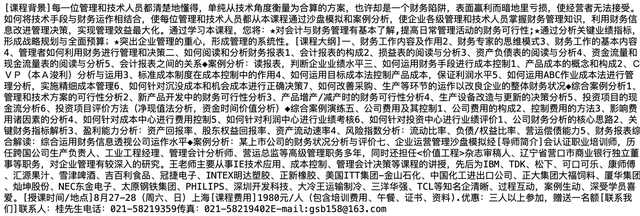`词性表: Ag 形语素 形容词性语素。形容词代码为 a，语素代码ｇ前面置以A。 a 形容词 取英语形容词 adjective的第1个字母。 ad 副形词 直接作状语的形容词。形容词代码 a和副词代码d并在一起。 an 名形词 具有名词功能的形容词。形容词代码 a和名词代码n并在一起。 b 区别词 取汉字“别”的声母。 c 连词 取英语连词 conjunction的第1个字母。 dg 副语素 副词性语素。副词代码为 d，语素代码ｇ前面置以D。 d 副词 取 adverb的第2个字母，因其第1个字母已用于形容词。 e 叹词 取英语叹词 exclamation的第1个字母。 f 方位词 取汉字“方” g 语素 绝大多数语素都能作为合成词的“词根”，取汉字“根”的声母。 h 前接成分 取英语 head的第1个字母。 i 成语 取英语成语 idiom的第1个字母。 j 简称略语 取汉字“简”的声母。 k 后接成分 l 习用语 习用语尚未成为成语，有点“临时性”，取“临”的声母。 m 数词 取英语 numeral的第3个字母，n，u已有他用。 Ng 名语素 名词性语素。名词代码为 n，语素代码ｇ前面置以N。 n 名词 取英语名词 noun的第1个字母。 nr 人名 名词代码 n和“人(ren)”的声母并在一起。 ns 地名 名词代码 n和处所词代码s并在一起。 nt 机构团体 “团”的声母为 t，名词代码n和t并在一起。 nz 其他专名 “专”的声母的第 1个字母为z，名词代码n和z并在一起。 o 拟声词 取英语拟声词 onomatopoeia的第1个字母。 p 介词 取英语介词 prepositional的第1个字母。 q 量词 取英语 quantity的第1个字母。 r 代词 取英语代词 pronoun的第2个字母,因p已用于介词。 s 处所词 取英语 space的第1个字母。 tg 时语素 时间词性语素。时间词代码为 t,在语素的代码g前面置以T。 t 时间词 取英语 time的第1个字母。 u 助词 取英语助词 auxiliary vg 动语素 动词性语素。动词代码为 v。在语素的代码g前面置以V。 v 动词 取英语动词 verb的第一个字母。 vd 副动词 直接作状语的动词。动词和副词的代码并在一起。 vn 名动词 指具有名词功能的动词。动词和名词的代码并在一起。 w 标点符号 x 非语素字 非语素字只是一个符号，字母 x通常用于代表未知数、符号。 y 语气词 取汉字“语”的声母。 z 状态词 取汉字“状”的声母的前一个字母。 un 未知词 不可识别词及用户自定义词组。取英文Unkonwn首两个字母。(非北大标准，CSW分词中定义)`
`i = 0 for w in pseg.cut(content_list[i], HMM=False):     print('%s' % (w.word),'%s' % (w.flag),end = ' ') print("\n"+"*"*70) delete_list = ['eng','m'] for w in pseg.cut(content_list[i], HMM=False):     if (w.flag not in delete_list and len(w.word)!=1) and w.word not in stop_word_list:         print('%s' % (w.word),end = ' ')     #if w.flag == 'm':     #    print('%s' % (w.word),end = ' ')`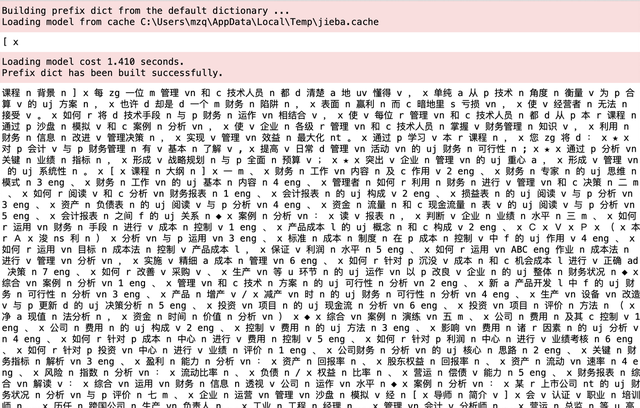`def get_word(content):     delete_list = ['eng','m']     result = []     for w in pseg.cut(content, HMM=False):         if (w.flag not in delete_list and len(w.word)!=1) and w.word not in stop_word_list:             result.append(w.word)     return result`
`cut_word_list = [] for i in tqdm(content_list):     cut_word_list.append(get_word(i)) #将切分之后的结果添加到列表中`
`data_result = pd.DataFrame(`
`data_result['分词结果'] = cut_word_list data_result['样本标签'] = label_list data_result.head(5)`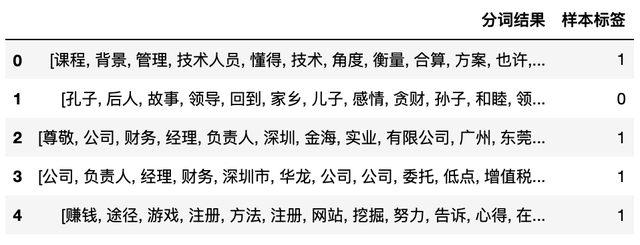`true_data = data_result[data_result['样本标签'] == 1]['分词结果'] false_data = data_result[data_result['样本标签'] == 0]['分词结果'] true_data`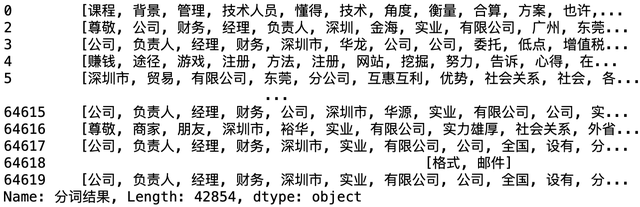`merge=itertools.chain.from_iterable(true_data) true_word = list(merge) merge=itertools.chain.from_iterable(false_data) false_word = list(merge) cloud_text_true = ",".join(true_word) cloud_text_false = ",".join(false_word) del true_word del false_word del true_data del false_data wc_true = WordCloud(     font_path="HGFS_CNKI.TTF",     background_color="white",     max_words=2000) # generate word cloud wc_false = WordCloud(     font_path="HGFS_CNKI.TTF",     background_color="white",     max_words=2000) # generate word cloud wc_false.generate(cloud_text_false) wc_true.generate(cloud_text_true) del cloud_text_false del cloud_text_true plt.figure(figsize=(15,5)) plt.subplot(1,2,1) plt.imshow(wc_true) plt.subplot(1,2,2) plt.imshow(wc_false)`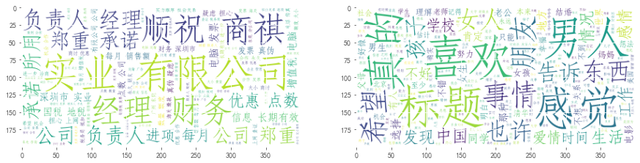`content_text = [' '.join(text) for text in cut_word_list] cv = CountVectorizer(max_features=5000,max_df=0.6,min_df=5) counts = cv.fit_transform(content_text)`
`tfidf = TfidfTransformer() tfidf_matrix = tfidf.fit_transform(counts)`
`import time import seaborn as sns train_X, test_X, train_y, test_y = train_test_split(tfidf_matrix, label_list, test_size=0.2,random_state=0) mnb = MultinomialNB() startTime = time.time() mnb.fit(train_X, train_y)  #训练过程 print('多项式贝叶斯分类器训练用时%.2f秒' %(time.time()-startTime)) sc1 = mnb.score(test_X, test_y)  #在测试集上计算得分 print('多项式贝叶斯分类器准确率为:',sc1) y_pred1 = mnb.predict(test_X) print('多项式贝叶斯分类器召回率为:', recall_score(test_y, y_pred1 )) def plot(matrix):     sns.set()     f,ax=plt.subplots()     print(matrix) #打印出来看看     sns.heatmap(matrix,annot=True,cmap="Blues",ax=ax,fmt='.20g') #画热力图     ax.set_title('confusion matrix') #标题     ax.set_xlabel('predict') #x轴     ax.set_ylabel('true') #y轴 plot(confusion_matrix(test_y,y_pred1))`